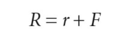# Fisher effect

(redirected from Fisher hypothesis)
Also found in: Wikipedia.

## Fisher effect

A theory that nominal interest rates in two or more countries should be equal to the required real rate of return to investors plus compensation for the expected amount of inflation in each country.

## Fisher Effect

A theory stating that real interest rates are independent of monetary considerations. According to the Fisher effect, a currency's real interest rate is equal to its nominal interest rate less the inflation rate. Thus, if the inflation rate rises, the real interest rate eventually rises as well; likewise, if inflation falls, the real interest rate will fall. See also: International Fisher Effect.

## Fisher effect

The direct relationship between inflation and interest rates. Increasing inflationary expectations result in increasing interest rates.

## Fisher effect

an expression that formally allows for the effects of INFLATION upon the INTEREST RATE of a LOAN or BOND. The Fisher equation, devised by Irving Fisher (1867–1947), expresses the nominal interest rate on a loan as the sum of the REAL INTEREST RATE and the rate of inflation expected over the duration of the loan:where R = nominal interest rate, r = real interest rate and F = rate of annual inflation. For example, if inflation is 6% in one year and the real interest rate required by lenders is 4%, then the nominal interest rate will be 10%. The inflation premium of 6% incorporated in the nominal interest rate serves to compensate lenders for the reduced value of the currency loaned when it is returned by borrowers.

The Fisher effect suggests a direct relationship between inflation and nominal interest rates, changes in annual inflation rates leading to matching changes in nominal interest rates. See INTERNATIONAL FISHER EFFECT.

Collins Dictionary of Economics, 4th ed. © C. Pass, B. Lowes, L. Davies 2005
References in periodicals archive ?
(2012) tested the validity of Fisher Hypothesis for Turkey for the period 1989:Q1 - 2011:Q4 using Johansen cointegration analysis.
'On the Pitfalls of Untested Common-Factor Restrictions: the Case of the Inverted Fisher Hypothesis', Oxford Bulletin of Economics and Statistics 50 (2): 125-38.
As Fisher Hypothesis (1930) concluded that nominal expected return on a security is a function of expected inflation rate as well as expected real interest rate.
Overview of the Fisher Hypothesis and the Empirical Mode
If the Fisher hypothesis holds, then short-term interest rates will be an efficient predictor of future inflation (Granville and Mallick, 2004).
However, this so-called generalized Fisher hypothesis has received little empirical support.
Second, the rejection of a unit root in the short-run interest rates sheds some light on the empirical investigation of two major relationships in macroeconomics--the Fisher Hypothesis (FH) and the Uncovered Interest Parity (UIP).
The Fisher hypothesis suggests that the nominal yield on conventional debt will compensate for anticipated inflation, thereby providing an acceptable real yield.
where If, [I.sup.future] is the actual future inflation rate, e is an error term with mean zero, and, if the Fisher hypothesis is correct and R is a constant, [Alpha] = -R and [Beta] = 1.
This estimate is insignificantly different from [1, -1] as implied by the Fisher hypothesis. Again, whether we account for these regime shifts or not, the unitary Fisher effect between inflation and municipal yields is maintained implying that the structural breaks are mean shifts not slope changes.
This article views the Fisher hypothesis as a long-run relationship with short-run variation in the real interest rate.

Site: Follow: Share:
Open / Close